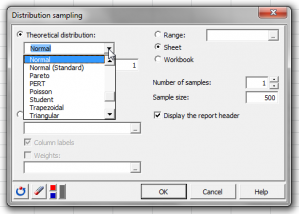# Distribution Sampling

Distribution sampling lets you generate small or large amounts of data from a theoretical distribution. Do it in Excel with the XLSTAT statistical software.## Distribution sampling: why?

From a dataset it may be useful to select a small or large amount of individuals to be submitted to further analyses. This can be used for validation purposes or to shorten the computation time.

Assuming you know the distribution of your sample you could generate a smaller or larger set that follows the same distribution to run your analyses and tests. You may enter your theoretical distribution parameters or let XLSTAT estimate them empirically from a given dataset.

## XLSTAT distribution sampling tool

This module generates random data based on a theoretical or empirical distribution. For a theoretical distribution, you must choose the probability distribution and define its parameters. For an empirical distribution, you must select a column with quantitative reference data.

XLSTAT provides the following distributions:

• Arcsine
• Bernoulli
• Beta (2 options)
• Binomial
• Negative binomial type I and II
• Chi-square
• Erlang
• Exponential
• Fisher
• Fisher-Tippett
• Gamma
• GEV (Generalized Extreme Values)
• Gumbel
• Logistic
• Lognormal (2 options)
• Normal
• Standard normal
• Pareto
• PERT
• Poisson
• Student
• Trapezoidal
• Triangular
• Uniform and Uniform discrete
• Weibull (3 options)### analyze your data with xlstat

14-day free trial

Included in

Related features